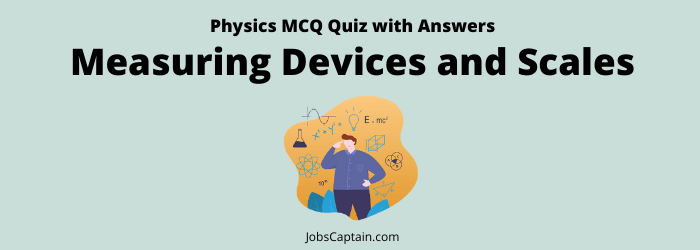# Physics: Measuring Devices and Scales MCQ Quiz 1Quiz Set 1 2

Question 1.  In SONAR, we use?

(A) Infrasonic waves

(B) Ultrasonic waves

(C) Audible sound waves

(B) Ultrasonic waves

Question 2.  The apparatus used to measure intensity of sound is ______.

(A) Audiometer

(B) Audiophone

(C) Anemeter

(D) Chronometer

(A) Audiometer

Question 3.  Which one of the following instrument is used for locating submerged objects in an ocean?

(A) SONAR

(B) Sextant

(C) Galvanometer

(D) Audiometer

(A) SONAR

Question 4. SONAR is mostly used by ________.

(A) Navigators

(B) Engineers

(C) Doctors

(D) Astronauts

(A) Navigators

Question 5. Which of the following is measured by ‘Anemometer’?

(A) Intensity of light

(B) Force of the wind

(C) Depth of water

(D) Velocity of water-flow

(B) Force of the wind

Question 6. The velocity of wind is measured by ________.

(A) Wind Vane

(B) Hydrometer

(C) Anemometer

(D) Barometer

(C) Anemometer

Question 7. Which one of the following is not correctly matched?

(A) Pyrometer – High temp

(B) Tachometer – Pressure difference

(C) Ammeter – Electric current

(D) Anemometer – Wind speed

(B) Tacheometer – Pressure difference

Question 8. Pyrometer is used to measure?

(A) Density

(B) High temperature

(C) Humidity

(D) Air pressure

(B) High temperature

Question 9. Which one of the following devices is used to measure extremely high temperature?

(A) Pycnometer

(B) Phonometer

(C) Photometer

(D) Pyrometer

(D) Pyrometer

Question 10. Which one of the following thermometers is known as pyrometer?

(A) Liquid thermometers

(B) Gas thermometers

(D) Thermo-electric thermometers

Question 11. Which of the following is correctly matched?

(A) Junction Diode – Amplifier

(B) Bipolar Junction Transistor – Rectifier

(C) Capacitor – Thermometer

(D) Thermoresistor – Electronic Thermometer

(D) Thermoresistor – Electronic Thermometer

Question 12. Hygrometer is used to measure?

(A) Velocity of wind

(B) High temperature

(C) Atmospheric pressure

(D) Humidity in atmosphere

(D) Humidity in atmosphere

Question 13. Which instrument is used for measuring humidity in the air?

(A)  Eudiometer

(B) Spectrometer

(C) Hygrometer

(D) Hydrometer

(C) Hygrometer

Question 14. The density of milk can be obtained by the use of?

(A) Thermometer

(B) Lactometer

(C) Butyrometer

(D) Hydrometer

(B) Lactometer

Question 15. The liquid/liquids used in the simple barometer is/are?
(A) Mercury

(B) Water

(C) Alcohol

(D)  All of these

(B) Water

Question 16. Which instrument is used to measure atmospheric pressure?

(A) Hygrometer

(B) Manometer

(C) Barometer

(D) Hydrometer

(C) Barometer

Question 17. Which one of the following pair is not correctly matched?

(A) Ammeter – Measuring instrument for electric power

(B) Audiometer – Device for measuring sound intensity

(C) Ondometer – Measuring instrument for frequency of electromagnetic waves

(D) Odometer – Measuring instrument for distance covered by motor wheels

(A) Ammeter – Measuring instrument for electric power

Question 18. What is measured by manometer?

(A) Pressure of oil on the surface

(B) Density of liquids

(C) Pressure of gas

(D) Air pressure

(C) Pressure of gas

Question 19. The thermometer which used to measure 2000ºC temperature is?

(A) Steam pressure thermometer

(C) Mercury thermometer

(D) Gas thermometer

Question 20. ‘Pyrheliometer’ is used for measuring?

(A) Temperature of plants

(B) Air temperature